# SSC Quantitative Aptitude Questions (Day-61)

Dear Aspirants, you can find the Quantitative Aptitude questions with detailed explanations for the SSC exams. Nowadays the competitive level of the exam has been increasing consistently. Due to the great demand for the government job, the level of the toughness reached greater. Candidates have to enhance the preparation process in order to drive in the right path. It doesn’t need to clear the prescribed cutoff. You must have to score good marks more than the cut off marks to get into the final provisional list. Here we have updating the Quantitative Aptitude questions with detailed explanations on a daily basis. You can practice with us and measure your level of preparation. According to that you can sculpt yourself in a proper way. SSC aspirants kindly make use of it and grab your success in your career.

Start Quiz

1) A certain sum becomes 5 times in 3 years, at simple interest, then in how many years it will become 15 times?

(a) 6

(b) 15

(c) 9

(d) 12

Direction (2-5): The line chart given below represents the runs scored by Kohli and Sharma against 5 teams.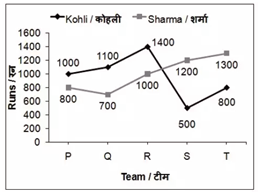2) What is the total runs scored by Kohli against 5 teams?

(a) 5000

(b) 4800

(c) 4700

(d) 4500

3) What is the difference between the total runs scored by Kohli against the teams Q and R and total runs scored by Sharma against the teams Q and R?

(a) 200

(b) 400

(c) 900

(d) 800

4) Runs scored by Kohli against team S is how much percent less than the runs scored by Sharma against team S?

70.21

68.33

58.33

41.66

5) Total runs scored by Sharma against 5 teams is what percent of total runs scored by Kohli against 5 teams?

(a) 104.16

(b) 96

(c) 98.13

(d) 108.24

6) If the height of a given cone becomes four times and the radius of the base becomes twice, then what is the ratio of the volume of the given cone and the volume of the new cone?

(a) 1 : 4

(b) 1 : 8

(c) 1 : 16

(d) 1 : 32

7) Parallel sides of a trapezium are 26 cm and 40 cm and the area is 792 cm2. What is the value of the distance (in cm) between parallel sides?

(a) 24

(b) 48

(c) 12

(d) 36

8) The area of a circle is same as the area of a square. What is the ratio of the diameter of the circle and diagonal of the square?

(a) 1 : √π

(b) 2 : √π

(c) √2 : √π

(d) 1 : π

9) If the perimeter of a rhombus is 40 cm and one of its diagonal is 16 cm, then what is the area (in cm2) of the rhombus?

(a) 72

(b) 48

(c) 96

(d) 192

10) Two identical circles intersects so that their centres and the points at which they intersect form a square of side 2 cm. What is the area (in cm2) of the portion that is common to the two circles?

(a) 2π – 4

(b) 4π – 8

(c) 3π – 4

(d) π – 2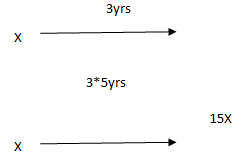15 times = 3 + 3 + 3 = 9 years

Total run scored by Kohli

= 1000 + 1100 + 1400 + 500 + 800

= 4800

Total run scored by Kohli against teams Q & R = 2500

Total runs scored by Sharma against teams Q & R = 1700

Difference = 2500 – 1700

= 800

Runs scored by Kohli against S = 500

Runs scored by Sharma against S = 1200

% less score = (1200 – 500)/1200 × 100

= 700/1200 × 100

= 58.33%

Total run scored by Sharma = 5000

Total run scored by Kohli = 4800

% score of Sharma with respect to Kohli

= 5000/4800 × 100

= 104.16%

Hnew = 4h

Rnew = 2r

Voriginal= 1/3 πr2 h

Vnew= 1/3 π 4r²4h

=16.1/3 πr2 h

Hence,

Voriginal :Vnew = 1 : 16

Area of trapezium = 1/2 (sum of parallel sides) × height

792 =  ½ ×(26 + 40) × height

Height = 24 cm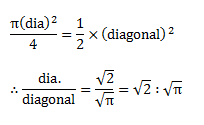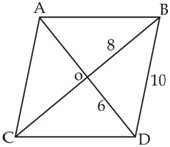If OB = 8 cm

&BD = 10 cm

thenOD = 6 cm

for Pythagoras theorem

Area of rhombus =1/2 d1×d2

= ½ × 16 × 12

= 96 cm²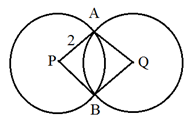Hence, radius of the circle = 2 cm

∵area of the common region = Area of sector – area of square

= 1/2 π × 4 – 4

=  2 – 4

 Check Here to View SSC CGL / CHSL 2021 Quantitative Aptitude Questions Day – 60 Day – 59 Day – 58